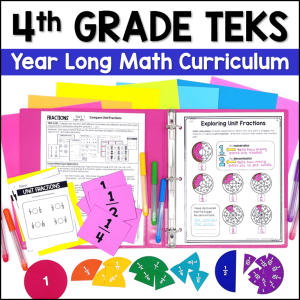Save 10% on your first order with code EXTRA10

# Calculating Profit in the Real World

\$3.00187.25\$22.00
SKU: 4025670 Category:

## Description

Your students will have fun practicing addition and subtraction of whole numbers and decimals with this activity. Students will Calculate Profit in each business scenario, and hopefully the entrepreneur inside of them will be inspired!

The 24 task cards are divided into 4 sets. Each set includes 6 task cards.

Use the sets to differentiate, or use them throughout the year to teach and review.

Profit at Fiesta: Problems with 2 and 3 digit whole numbers; situations give 2 expenses

Profit at the Market: Problems with decimals and whole numbers; situations give 2 or 3 expenses. Students must add a decimal point and trailing zeros to add and subtract

Profit at the Bakery: Problems with decimals and whole numbers; situations give 3 expenses; some have extra information; students must add a decimal point and trailing zeros to subtract

Profit at the Office: Problems with decimals and whole numbers; whole numbers are up to 5 digits; students must add a decimal point and trailing zeros to subtract.

TEKS Alignment:

4.4A Add and subtract whole numbers and decimals to the hundredths place using the standard algorithm

4.10B Calculate profit in a given situation